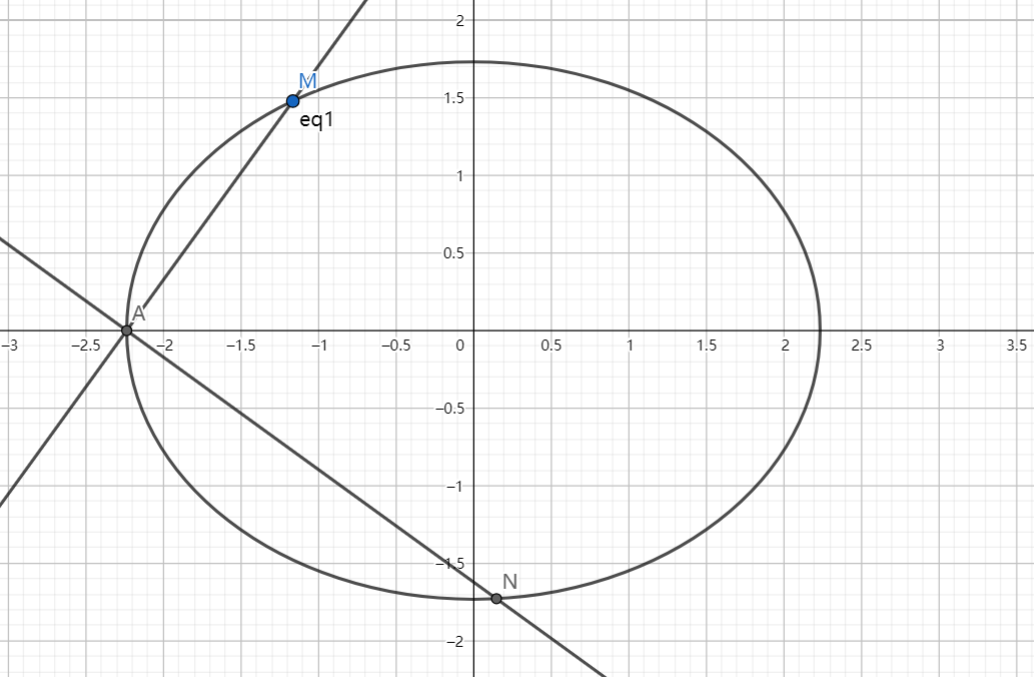# SAT1000 - P811

Geometry Level pendingAs shown above, given that ellipse $E: \dfrac{x^2}{t}+\dfrac{y^2}{3}=1\ (t>3)$, point $A$ is the left vertex of $E$, and line $l$ whose slope is $k\ (k>0)$ intersects with $E$ at point $A,M$, point $N$ is a point on $E$ such that $MA \perp NA$.

If $2|AM|=|AN|$, find the range of $k$ as $t$ changes.

If the range can be expressed as $(l,r)$, submit $\lfloor 1000(2r-l) \rfloor$.

Have a look at my problem set: SAT 1000 problems

×